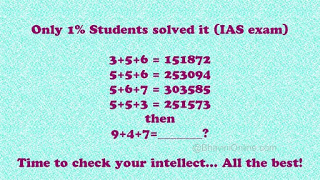## Monday, 21 December 2020

Hello friends how are you and today I have come up with a good and fun post for you and below is all the information

You will also share the answer below and it will be fun to share this information with your friends and also the information of this work and give in the area below and comment if any information is left.The solution to the first 4 digits is pretty straight forward, and I am sure most of you must have easily got it.

It works out to be a+b+c = (a*b)(a*c)??
The last 2 digits are really tough to crack…
Here’s how you do it. Reverse of {(a×b)+(a×c)-c}
So the number would (a×b),(a×c),{(a×b)+(a×c)-c}reverse.
So for 1st one: 3+5+6=151872;
axb = 3×5 = 15; axc = 3×6 = 18;
{(a×b)+(a×c)-c} = ((3×5)+(3×6)-6} = 27
— Reverse of 27 is 72
So 9+4+7=_______?
axb = 9×4 = 36;
axc = 9×7 = 63;
{(a×b)+(a×c)-c} = ((9×4)+(9×7)-7} = (36+63-7)=92
— Reverse of 92 is 29.

Answer : Hence 9+4+7 = 366329

You will find very good information to learn and learn from this web site. You will also find all the information and material to learn here. Share more and more.